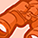# New to Qlik Sense

If you’re new to Qlik Sense, start with this Discussion Board and get up-to-speed quickly.

Announcements
Qlik Insider: SaaS capabilities for Data Integration and Analytics, May 25th! Register Now
cancel
Showing results for
Did you mean:Contributor III

## Date comparison in set expressions

Hey everyone,

I have been trying to implement a set expression for my bar chart, however I am not being able to put the formula in a correct way to present the correct data on my board.

So, I have Months as dimension and Invoice Amount as measure for this bar chart.

I need to filter the measure on 3 other columns, and am trying the below:

Sum({\$<[Overall  Status]={'No'}>*<[Class]={'Sundry'}>*<[Invoice_Date]={"\$(<today())"}>}[Invoice Amount])

When I filter on first two expressions, the plot shows results, but when I include the third the plot is empty.

Thanks!

1 Solution

Accepted SolutionsSpecialist III

Hello Edil,

Please try below given sample expression:

Sum({\$<[Overall  Status]={'No'}, [Class]={'Sundry'}, [Invoice_Date]={"<\$(=Today())"}>}[Invoice Amount])

Regards!

Rahul

6 RepliesCreator

Hi,

Try this,

Sum({<[Overall  Status]={'No'},[Class]={'Sundry'},[Invoice_Date]={"\$(<today())"}>}[Invoice Amount])Contributor III
Author

Same result.

Just to make it clearer for some:

I also created a separate table or another graph with a measure or dimension defined by:

if(Invoice_Date-today()<0,'Overdue','Due')

it works fine, but with the Sum set expression, i am facing the problem.Creator III

can you please post the sample appSpecialist III

Hello Edil,

Please try below given sample expression:

Sum({\$<[Overall  Status]={'No'}, [Class]={'Sundry'}, [Invoice_Date]={"<\$(=Today())"}>}[Invoice Amount])

Regards!

RahulContributor III
Author

Thank you Rahul,

You are the guru!

This was helpful, I used what u suggested, however I do not understand what is the syntax trick here. What is the sequence that needs to be followed?Specialist III

Hello Edil,

Thank you for your appreciation. We are branches of same Tree.

In above example we would like to calculate Total Invoice Amount in the context of Overall Status, Class and Invoice Date. If you break the expression into parts then I will broadly categories them as- Identifier, Modified and Measure field.

Step 1:

// Get the Total Invoice Amount

Sum([Invoice Amount])

Step 2:

// Get the Total Invoice Amount with Identifier i.e. current selection

Sum({\$}[Invoice Amount])

Step 3:

// Get the Total Invoice Amount where Overall Status is No in current selection context

Sum({\$<[Overall  Status]={'No'}>}[Invoice Amount])

Step 4:

// Get the Total Invoice Amount where Overall Status is No and Class is Sundry in current selection context

Sum({\$<[Overall  Status]={'No'}, [Class]={'Sundry'}>}[Invoice Amount])

Step 5:

// Get the Total Invoice Amount where Overall Status is No, Class is Sundry and Invoice Date is less than today's date in current selection context

Sum({\$<[Overall  Status]={'No'}, [Class]={'Sundry'}, [Invoice_Date]={"<\$(=Today())">}[Invoice Amount])

Note: Here we used \$ sign expansion to evaluate the value for Today function. This result is feed to Invoice Date with Less than operator within the quotes.

We can use multiple conditions for single Identifier.

For example,

sum( {\$<Year = {“>1978<2004”}>} Sales )

This will returns the sales for the current selections, but with a numeric search used to scope the range of years to sum the sales across i.e. sales between 1979 & 2003.

Hope this will make sense.

Regards!

RahulTags